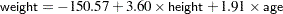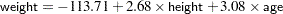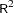Previous Page | Next Page

 The REG Procedure

## Example 74.3 Predicting Weight by Height and Age

In this example, the weights of schoolchildren are modeled as a function of their heights and ages. The example shows the use of a BY statement with PROC REG, multiple MODEL statements, and the OUTEST= and OUTSSCP= options, which create data sets. Here are the data:

```*------------Data on Age, Weight, and Height of Children-------*
| Age (months), height (inches), and weight (pounds) were      |
| recorded for a group of school children.                     |
| From Lewis and Taylor (1967).                                |
*--------------------------------------------------------------*;

data htwt;
input sex \$ age :3.1 height weight @@;
datalines;
f 143 56.3  85.0 f 155 62.3 105.0 f 153 63.3 108.0 f 161 59.0  92.0
f 191 62.5 112.5 f 171 62.5 112.0 f 185 59.0 104.0 f 142 56.5  69.0
f 160 62.0  94.5 f 140 53.8  68.5 f 139 61.5 104.0 f 178 61.5 103.5
f 157 64.5 123.5 f 149 58.3  93.0 f 143 51.3  50.5 f 145 58.8  89.0

... more lines ...

m 164 66.5 112.0 m 189 65.0 114.0 m 164 61.5 140.0 m 167 62.0 107.5
m 151 59.3  87.0
;
```

Modeling is performed separately for boys and girls. Since the BY statement is used, interactive processing is not possible in this example; no statements can appear after the first RUN statement.

The following statements produce Output 74.3.1 through Output 74.3.4:

```proc reg outest=est1 outsscp=sscp1 rsquare;
by sex;
eq1: model  weight=height;
eq2: model  weight=height age;

proc print data=sscp1;
title2 'SSCP type data set';

proc print data=est1;
title2 'EST type data set';
run;
```

Output 74.3.1 Height and Weight Data: Submodel for Female Children
The REG Procedure
Model: eq1
Dependent Variable: weight

Analysis of Variance
Source DF Sum of
Squares
Mean
Square
F Value Pr > F
Model 1 21507 21507 141.09 <.0001
Error 109 16615 152.42739
Corrected Total 110 38121

 Root MSE R-Square 12.3461 0.5642 98.8784 0.5602 12.4862

Parameter Estimates
Variable DF Parameter
Estimate
Standard
Error
t Value Pr > |t|
Intercept 1 -153.12891 21.24814 -7.21 <.0001
height 1 4.16361 0.35052 11.88 <.0001

Output 74.3.2 Height and Weight Data: Full Model for Female Children
The REG Procedure
Model: eq2
Dependent Variable: weight

Analysis of Variance
Source DF Sum of
Squares
Mean
Square
F Value Pr > F
Model 2 22432 11216 77.21 <.0001
Error 108 15689 145.26700
Corrected Total 110 38121

 Root MSE R-Square 12.0527 0.5884 98.8784 0.5808 12.1894

Parameter Estimates
Variable DF Parameter
Estimate
Standard
Error
t Value Pr > |t|
Intercept 1 -150.59698 20.76730 -7.25 <.0001
height 1 3.60378 0.40777 8.84 <.0001
age 1 1.90703 0.75543 2.52 0.0130

Output 74.3.3 Height and Weight Data: Submodel for Male Children
The REG Procedure
Model: eq1
Dependent Variable: weight

Analysis of Variance
Source DF Sum of
Squares
Mean
Square
F Value Pr > F
Model 1 31126 31126 206.24 <.0001
Error 124 18714 150.92222
Corrected Total 125 49840

 Root MSE R-Square 12.285 0.6245 103.448 0.6215 11.8755

Parameter Estimates
Variable DF Parameter
Estimate
Standard
Error
t Value Pr > |t|
Intercept 1 -125.69807 15.99362 -7.86 <.0001
height 1 3.68977 0.25693 14.36 <.0001

Output 74.3.4 Height and Weight Data: Full Model for Male Children
The REG Procedure
Model: eq2
Dependent Variable: weight

Analysis of Variance
Source DF Sum of
Squares
Mean
Square
F Value Pr > F
Model 2 32975 16487 120.24 <.0001
Error 123 16866 137.11922
Corrected Total 125 49840

 Root MSE R-Square 11.7098 0.6616 103.448 0.6561 11.3194

Parameter Estimates
Variable DF Parameter
Estimate
Standard
Error
t Value Pr > |t|
Intercept 1 -113.71346 15.59021 -7.29 <.0001
height 1 2.68075 0.36809 7.28 <.0001
age 1 3.08167 0.83927 3.67 0.0004

For both female and male children, the overallstatistics for both models are significant, indicating that the model explains a significant portion of the variation in the data. For females, the full model isand for males, the full model isThe OUTSSCP= data set is shown in Output 74.3.5. Note how the BY groups are separated. Observations with _TYPE_=‘N’ contain the number of observations in the associated BY group. Observations with _TYPE_=‘SSCP’ contain the rows of the uncorrected sums of squares and crossproducts matrix. The observations with _NAME_=‘Intercept’ contain crossproducts for the intercept.

Output 74.3.5 SSCP Matrix
Obs sex _TYPE_ _NAME_ Intercept height weight age
1 f SSCP Intercept 111.0 6718.40 10975.50 1824.90
2 f SSCP height 6718.4 407879.32 669469.85 110818.32
3 f SSCP weight 10975.5 669469.85 1123360.75 182444.95
4 f SSCP age 1824.9 110818.32 182444.95 30363.81
5 f N   111.0 111.00 111.00 111.00
6 m SSCP Intercept 126.0 7825.00 13034.50 2072.10
7 m SSCP height 7825.0 488243.60 817919.60 129432.57
8 m SSCP weight 13034.5 817919.60 1398238.75 217717.45
9 m SSCP age 2072.1 129432.57 217717.45 34515.95
10 m N   126.0 126.00 126.00 126.00

The OUTEST= data set is displayed in Output 74.3.6; again, the BY groups are separated. The _MODEL_ column contains the labels for models from the MODEL statements. If no labels are specified, the defaults MODEL1 and MODEL2 would appear as values for _MODEL_. Note that _TYPE_=‘PARMS’ for all observations, indicating that all observations contain parameter estimates. The _DEPVAR_ column displays the dependent variable, and the _RMSE_ column gives the root mean square error for the associated model. The Intercept column gives the estimate for the intercept for the associated model, and variables with the same name as variables in the original data set (height, age) give parameter estimates for those variables. The dependent variable, weight, is shown with a value of. The _IN_ column contains the number of regressors in the model not including the intercept; _P_ contains the number of parameters in the model; _EDF_ contains the error degrees of freedom; and _RSQ_ contains thestatistic. Finally, note that the _IN_, _P_, _EDF_, and _RSQ_ columns appear in the OUTEST= data set since the RSQUARE option is specified in the PROC REG statement.

Output 74.3.6 OUTEST Data Set
Obs sex _MODEL_ _TYPE_ _DEPVAR_ _RMSE_ Intercept height weight age _IN_ _P_ _EDF_ _RSQ_
1 f eq1 PARMS weight 12.3461 -153.129 4.16361 -1 . 1 2 109 0.56416
2 f eq2 PARMS weight 12.0527 -150.597 3.60378 -1 1.90703 2 3 108 0.58845
3 m eq1 PARMS weight 12.2850 -125.698 3.68977 -1 . 1 2 124 0.62451
4 m eq2 PARMS weight 11.7098 -113.713 2.68075 -1 3.08167 2 3 123 0.66161Previous Page | Next Page | Top of Page